Collection |

Statistics for Biologists

There is no disputing the importance of statistical analysis in biological research, but too often it is considered only after an experiment is completed, when it may be too late.

This collection highlights important statistical issues that biologists should be aware of and provides practical advice to help them improve the rigor of their work.

Nature Methods' Points of Significance column on statistics explains many key statistical and experimental design concepts. Other resources include an online plotting tool and links to statistics guides from other publishers.

Image Credit: Erin DeWalt

Since September 2013 Nature Methods has been publishing a monthly column on statistics called "Points of Significance." This column is intended to provide reseachers in biology with a basic introduction to core statistical concepts and methods, including experimental design. Although targeted at biologists, the articles are useful guides for researchers in other disciplines as well. A continuously updated list of these articles is provided below.Importance of being uncertain - How samples are used to estimate population statistics and what this means in terms of uncertainty.Error Bars - The use of error bars to represent uncertainty and advice on how to interpret them.Significance, P values and t-tests - Introduction to the concept of statistical significance and the one-sample t-test.Power and sample size - Use of statistical power to optimize study design and sample numbers.Visualizing samples with box plots - Introduction to box plots and their use to illustrate the spread and differences of samples. See also: Kick the bar chart habit and BoxPlotR: a web tool for generation of box plotsComparing samples—part I - How to use the two-sample t-test to compare either uncorrelated or correlated samples.Comparing samples—part II - Adjustment and reinterpretation of P values when large numbers of tests are performed.Nonparametric tests - Use of nonparametric tests to robustly compare skewed or ranked data.Designing comparative experiments - The first of a series of columns that tackle experimental design shows how a paired design achieves sensitivity and specificity requirements despite biological and technical variability.Analysis of variance and blocking - Introduction to ANOVA and the importance of blocking in good experimental design to mitigate experimental error and the impact of factors not under study.Replication - Technical replication reveals technical variation while biological replication is required for biological inference.Nested designs - Use the relative noise contribution of each layer in nested experimental designs to optimally allocate experimental resources using ANOVA.Two-factor designs - It is common in biological systems for multiple experimental factors to produce interacting effects on a system. A study design that allows these interactions can increase sensitivity.Sources of variation - To generalize experimental conclusions to a population, it is critical to sample its variation while using experimental control, randomization, blocking and replication for replicable and meaningful results.Split plot design - When some experimental factors are harder to vary than others, a split plot design can be efficient for exploring the main (average) effects and interactions of the factors.Bayes’ theorem - Use Bayes’ theorem to combine prior knowledge with observations of a system and make predictions about it.Bayesian statistics - Unlike classical frequentist statistics, Bayesian statistics allows direct inference of the probability that a model is correct and it provides the ability to update this probability as new data is collected.Sampling distributions and the bootstrap - Use the bootstrap method to simulate new samples and assess the precision and bias of sample estimates.Bayesian networks - Model interactions between causes and effects in large networks of causal influences using Bayesian networks, which combine network analysis with Bayesian statistics.Association, correlation and causation - Pairwise dependencies can be characterized using correlation but be aware that correlation only implies association, not causation. Conversely, causation implies association, not correlation.Simple linear regression - Given data on the relationship between two variables, linear regression is a simple and surprisingly robust method to predict unknown values.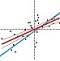Multiple linear regression - When multiple variables are associated with a response, the interpretation of a            prediction equation is seldom simple.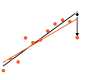Analyzing outliers: influential or nuisance - Some outliers influence the regression fit more than others.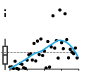Regression diagnostics - Residual plots can be used to validate assumptions about the regression model.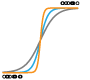Logistic regression - Regression can be used on categorical responses to estimate probabilities and to classify.Classification evaluation - It is important to understand both what a classification metric expresses and what it hides.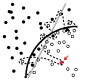Model selection and overfitting - "With four parameters I can fit an elephant and with five I can make him wiggle his trunk". John von Neumann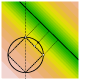Regularization - Constraining the magnitude of parameters of a model can control its complexity.P values and the search for significance - Little P value What are you tryign to say Of significance - Steve Ziliak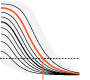Interpreting P values - A P value measures a sample's compatability with a hypothesis, not the truth of the hypothesis.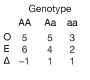Tabular data - Tabulating the number of objects in categories of interest dates back to the earliest records of commerce and population censuses.Clustering - Clustering finds patterns in data, whether they are there or not.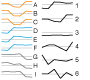Principal component analysis - PCA helps you interpret your data, but it will not always find the important patterns.Classification and regression trees - Decision trees are a simple but powerful prediction method.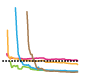Machine learning: a primer - Machine learning extracts patterns from data without explicit instructions.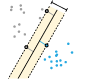Machine learning: supervised methods - Supervised learning algorithms extract general principles from observed examples guided by a specific prediction objective.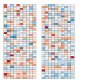Statistics versus machine learning - Statistics draws population inferences from a sample, and machine learning finds generalizable predictive patterns.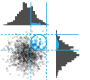The curse(s) of dimensionality - There is such a thing as too much of a good thing.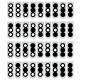Optimal experimental design - Customize the experiment for the setting instead of adjusting the setting to fit a classical design.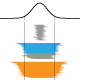Predicting with confidence and tolerance – For studying phenomena that are ‘typical’ rather than ‘average’.Two-level factorial experiments – Getting the benefits of probing multiple experimental factors at a time.